# Quiz 18: Activity-Based Costing

Activity Based Costing (ABC) : In traditional costing approach, costs are assigned to product or service. It is effective only when costs are direct costs. In the case of indirect costs, it is difficult to allocate costs. This problem is solved in Activity Based Costing as it allocate costs to activities involved in product or service rather than to product or service itself. In the given problem, W hospital was allocating all its maintenance costs to departments on the basis of departmental square feet. But now it is considering using ABC approach for cost allocation for maintenance cost related to repair. We need to calculate cost assigned to pharmacy department using both methods. Computation of Costs allocated to department on the basis of existing method is as follows: 1. Existing method : In this method, all costs are assigned on the basis of departmental square feet.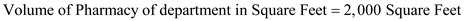Total labor hours for all departments having total volume of 100,000 square feet are 14,000 hours.The total labor cost per hour is \$13.71.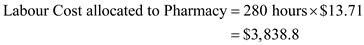Total supplies for all departments having total volume of 100,000 square feet are \$100,000.Total administration cost for all departments having total volume of 100,000 square feet are \$15,000.Computation of Total cost of Pharmacy department is as follows:Thus the value of total cost of pharmacy department has been computed above as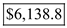. 2. ABC method : In this method, maintenance costs related to repair are assigned differently. For pharmacy department, labor hours for repair are 6 hours with cost of \$18 per hour. The other labor hours can be allocated on the basis of square feet which are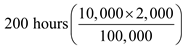. These labor hours have cost of \$12 per hour.For pharmacy department, supplies used for repair are \$200. The other supplies can be allocated on the basis of square feet which are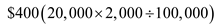.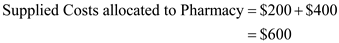In both the cases, administration costs shall remain same what it is in above method \$300. Computation of Total cost assigned to pharmacy department is as follows: